# Methods in Fit Binary Logistic Model

Select the method or formula of your choice.

## Factor/covariate pattern

Describes a single set of factor/covariate values in a data set. Minitab calculates event probabilities, residuals, and other diagnostic measures for each factor/covariate pattern.

For example, if a data set includes the factors gender and race and the covariate age, the combination of these predictors may contain as many different covariate patterns as subjects. If a data set only includes the factors race and sex, each coded at two levels, there are only four possible factor/covariate patterns. If you enter your data as frequencies, or as successes, trials, or failures, each row contains one factor/covariate pattern.

## Internal weights for Fit Binary Logistic Model

For generalized linear models, the weight matrix is not the identity matrix in cases where you do not specify weights. You can think of the internal weights as giving more influence on the analysis to observations with more data. For example, in fit binary logistic regression, the internal weight tends to be larger when the number of trials is larger.

### Formula

The following formulas give the internal weights for specific link functions:
Logit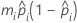Normit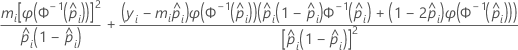Gompit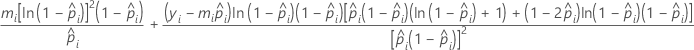### Notation

TermDescription
mithe number of trials for the ith row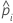the predicted probability for the design point in a binary logistic model
yithe number of events for the ith row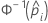the inverse cumulative distribution function of the standard normal distribution for the predicted probability in a binary logistic model

## How Minitab removes highly correlated predictors from the regression equation in Fit Binary Logistic Model

Let rij be the element in the current swept matrix associated with Xi and Xj.

Variables are entered or removed one at a time. Xk is eligible for entry if it is an independent variable not currently in the model with rkk ≥ 1 (tolerance with a default of 0.0001) and also for each variable Xj that is currently in the model,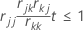To remove highly correlated predictors from a regression equation, Minitab does the following steps:
1. Minitab performs the SWEEP method on the correlation matrix, R, treating X1 … Xp as if they are random variables.
2. For any continuous predictor, Minitab compares the element rkk with the tolerance; rkk ≥ tolerance, where k = 1 to p.
3. For each variable Xj currently in the model, Minitab checks that (rjj – rjk * (rkj / rkk)) * tolerance ≤ 1.
###### Note

Where rkk, rjk, rjj are the corresponding diagonal and off diagonal elements for Xj and Xk variables after k step SWEEP operations.

4. Otherwise, the predictor fails the test and is removed from the model.
###### Note

The default tolerance value is 8.8e–12.

###### Note

You can use the TOLERANCE subcommand with the GZLM session command to force Minitab to keep a predictor in the model that is highly correlated with a different predictor. However, lowering the tolerance can be dangerous, possibly producing numerically inaccurate results.

By using this site you agree to the use of cookies for analytics and personalized content.  Read our policy